Science, Maths & Technology

### Become an OU student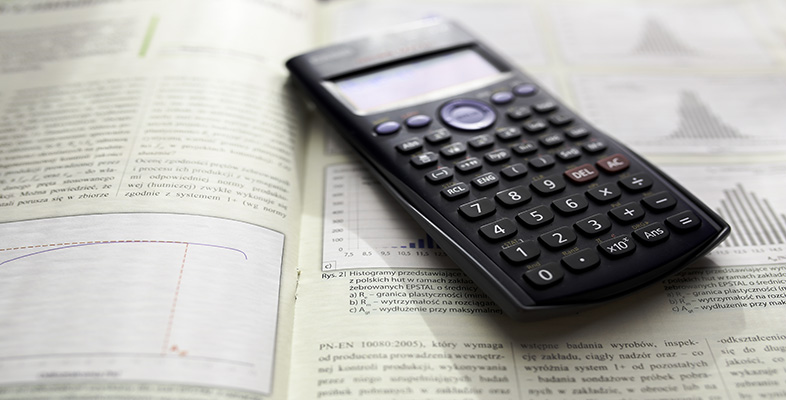Using a scientific calculator

Start this free course now. Just create an account and sign in. Enrol and complete the course for a free statement of participation or digital badge if available.

# 4 Doing longer calculations using your calculator

The volume of wood (in cubic metres) contained in a log of length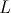metres with a distance around its middle of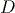metres is given by the formula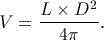For a log of length 1.5 m with a distance around the middle of 92 cm, this becomes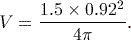In this section we consider several different approaches that can be used to evaluate this and other more complex expressions using different functions on your calculator. While the first method – considered in Activity 9 – is probably the most straightforward for this relatively simple expression, it is useful to see how you might use other calculator functions when you are faced with more complicated expressions to evaluate.

The expression for the volume of wood requires the value of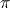. You could enter an approximate value forby hand, but this is time-consuming and may be prone to error. The calculator has an approximation forbuilt into it, which is obtained using the key sequence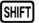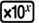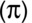.

Thekey is located on the bottom row of the keypad.

## Activity 9 Using the fraction key

The most obvious way of calculating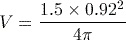is to enter it as a fraction on your calculator.

What key sequence is needed, and what is the final answer to 3 significant figures?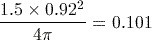(to 3 significant figures).

The key sequence used was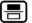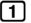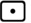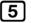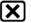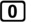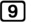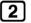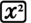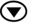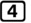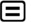.

Note that it is not strictly necessary to include the multiplication between the 4 and thein the denominator since if the sign is omitted, it will be assumed by the calculator.

Another way to carry out the calculation in Activity 9 is to use the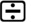key.

## Activity 10 Using thekey

You will not obtain the correct answer to the calculation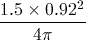if you type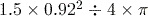into your calculator and press. Can you explain why? Insert a pair of brackets into the expression with the ÷ sign so that it will give the correct answer. Then type this new expression into the calculator and check that you obtain the same answer as in the activity above.

Typing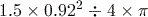into the calculator and pressingwill not give the correct answer because the calculator will follow the BIDMAS rules and divide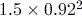by 4 and then multiply by, instead of dividing by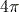.
To obtain the correct result, you have to type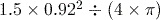. (Alternatively, you can type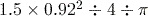.)